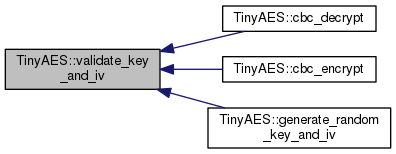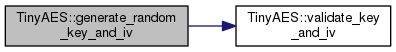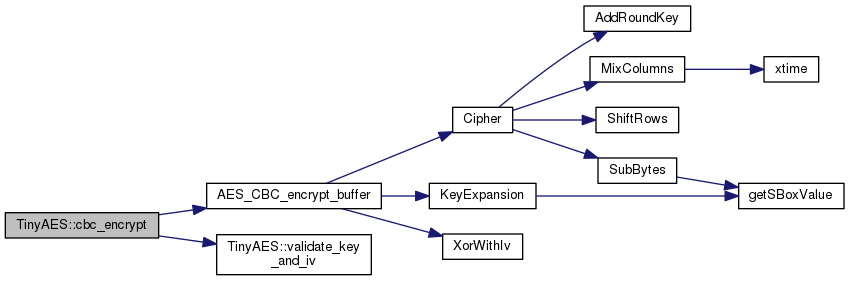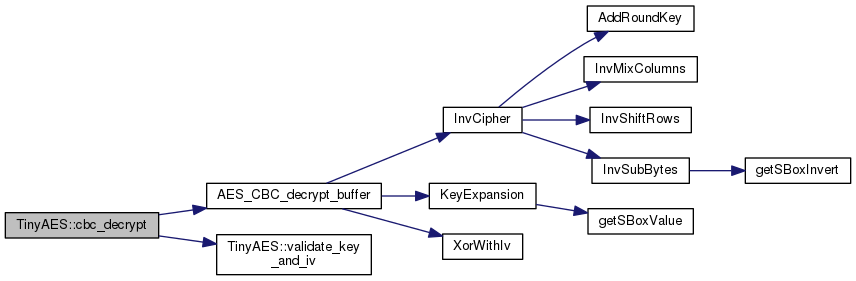TinyAES++  v0.0.1-2438 C++ API for TinyAESTinyAES Namespace Reference

TinyAES provides a few variants of the encryption/decryption methods in the AES family. More...

## Typedefs

typedef std::vector< uint8_t > VBytes
A vector of bytes. More...

## Functions

void validate_key_and_iv (const VBytes &key, const VBytes &iv)
Used internally to validate the length of both the key and the initialization vector. More...

void generate_random_key_and_iv (VBytes &key, VBytes &iv, size_t seed=0)
Generate a random 256-bit key and a 128-bit initialization vector. More...

std::string to_hex_string (const VBytes &v)
Convert the vector into a text string of hex characters. More...

VBytes from_hex_string (const std::string &str)
Convert the text string back into a vector of bytes. More...

std::string cbc_encrypt (const std::string &str, const VBytes &key, const VBytes &iv)
Encrypt the input buffer using 256-bit AES Cipher Block Chaining mode. More...

VBytes cbc_encrypt (const VBytes &input, const VBytes &key, const VBytes &iv)
Encrypt the input buffer using 256-bit AES Cipher Block Chaining mode. More...

std::string cbc_decrypt (const std::string &input, const VBytes &key, const VBytes &iv)
Decrypt the buffer using 256-bit AES Cipher Block Chaining mode. More...

VBytes cbc_decrypt (const VBytes &input, const VBytes &key, const VBytes &iv)
Decrypt the input buffer using 256-bit AES Cipher Block Chaining mode. More...

## Variables

const size_t key_size_in_bytes = 32
The number of bytes in a key, which is 32 for 256-bit AES. More...

const size_t iv_size_in_bytes = 16
The number of bytes in the initialization vector. More...

## Detailed Description

TinyAES provides a few variants of the encryption/decryption methods in the AES family.

Only 256-bit AES is enabled in this library, and only a few modes are available:

Note
The C++ wrapper only exposes 256-bit AES in CBC mode! No other bit size, and no other mode is enabled if you are using the TinyAES namespace from within your C++ application.

You need 3 things to encrypt and decrypt when using 256-bit AES in CBC mode:

• a 256-bit key, meaning 32 bytes of random data
• a 128-bit initialization vector, meaning 16 bytes of random data
• a text string or binary data that you want to encrypt and/or decrypt

The key and the iv (initialization vector) are required to correctly decrypt what was previously encrypted. If you don't have the key and the iv, you cannot decrypt. Attempting to guess the key and the iv is unlikely: with a 256-bit key, it would take a supercomputer trillions of years to crack the key via brute force exhaustion of the key space.

This library can help with the following tasks:

The encrypt/decrypt functions that take and return a `std::string` instead of a vector of bytes were added to make life easy for developers who happen to have some `std::string` objects they want encrypted. The API converts the strings to TinyAES::VBytes objects, and then converts the results back to `std::string`.

If wondering whether you should use the `std::string` or TinyAES::VBytes function calls, prefering TinyAES::VBytes will save two extra copies of the data – one on input, and the other on output.

In the simplest case, this is how you'd encrypt and decrypt a string of text:

#include <TinyAES.hpp>
// ...
std::string original_string = "Hello, World!";
std::string encrypted_string = TinyAES::cbc_encrypt(original_string, key, iv);
// ...
std::string decrypted_string = TinyAES::cbc_decrypt(encrypted_string, key, iv);

If you need to store and restore the 32-byte key and 16-byte initialization vector, this can easily be done using TinyAES::to_hex_string() and TinyAES::from_hex_string().

It is up to the application or the end user to ensure the key and iv are either copied or moved to an appropriate location to decrypt. There are no magical functions within TinyAES which will move the key and iv to their required final destination. And you cannot simply encrypt the key and the iv, since decryption requires you to have the key and iv!

## ◆ VBytes

 typedef std::vector TinyAES::VBytes

A vector of bytes.

Used to pass in the key, the initialization vector, and the byte to encrypt/decrypt.

#include <TinyAES.hpp>
using Vector_of_bytes=TinyAES::VBytes;
Vector_of_bytes get_iv()
{
// create and return a 128-bit (16 byte) initialization vector
Vector_of_bytes v =
{
// poor choice for an iv (consecutive bytes, perhaps easy to guess?)
// but shows how to easily instantiate a vector of bytes;
0x00, 0x01, 0x02, 0x03, 0x04, 0x05, 0x06, 0x07,
0x08, 0x09, 0x0a, 0x0b, 0x0c, 0x0d, 0x0e, 0x0f
};
return v;
}
Vector_of_bytes get_key()
{
// create and return a 256-bit (32 byte) encryption/decryption key
Vector_of_bytes v =
{
// same comment as above, non-random key would still encrypt/decrypt
// as expected, but attackers might have an easy time guessing the
0x00, 0x01, 0x02, 0x03, 0x04, 0x05, 0x06, 0x07,
0x08, 0x09, 0x0a, 0x0b, 0x0c, 0x0d, 0x0e, 0x0f,
0x10, 0x11, 0x12, 0x13, 0x14, 0x15, 0x16, 0x17,
0x18, 0x19, 0x1a, 0x1b, 0x1c, 0x1d, 0x1e, 0x1f
};
return v;
}

## ◆ validate_key_and_iv()

 void TinyAES::validate_key_and_iv ( const VBytes & key, const VBytes & iv )

Used internally to validate the length of both the key and the initialization vector.

Note
Under normal circumstances, user code has no need to make explicit calls to this function.
Exceptions
 std::length_error Throws if the key isn't exactly 32 bytes long. std::length_error Throws if the iv isn't exactly 16 bytes long.

References iv_size_in_bytes, and key_size_in_bytes.

Referenced by cbc_decrypt(), cbc_encrypt(), and generate_random_key_and_iv().

Here is the caller graph for this function:## ◆ generate_random_key_and_iv()

 void TinyAES::generate_random_key_and_iv ( VBytes & key, VBytes & iv, size_t seed = `0` )

Generate a random 256-bit key and a 128-bit initialization vector.

These will need to be stored somewhere, since decrypting requires the same key and iv as was used during encryption.

if (previous_app_settings_exist)
{
}
if (key.size() != TinyAES::key_size_in_bytes ||
{
// we *must* have a valid key and iv to encrypt/decrypt
save_encryption_key(key);
save_encryption_iv(iv);
}
Parameters
 [out] key Any previous content will be completely overwritten. On output, the key will contain exactly 256 bits (32 bytes). [out] iv Any previous content will be completely overwritten. On output, the initialization vector will contain exactly 128 bits (16 bytes). [in] seed If left as zero, a seed based on the current time will be used. If set to anything other than zero, that value will be used as a seed.

References iv_size_in_bytes, key_size_in_bytes, and validate_key_and_iv().

Here is the call graph for this function:## ◆ to_hex_string()

 std::string TinyAES::to_hex_string ( const VBytes & v )

Convert the vector into a text string of hex characters.

Useful in converting the encryption key and initialization vector to a text string.

std::cout << "key=" << TinyAES::to_hex_string(key) << std::endl;
std::cout << "iv=" << TinyAES::to_hex_string(iv) << std::endl;

The output would look similar to this:

key=ba531427ce62454abcbdfd37a247593d9dc141ccce55f4f978e831763325decd
iv=67bc931c150804b1ba2152b01b368b26

Note how the key is twice as long as the initialization vector, since the key contains 256 bits (32 bytes) of data and the iv contains 128 bits (16 bytes) of data.

from_hex_string()

## ◆ from_hex_string()

 TinyAES::VBytes TinyAES::from_hex_string ( const std::string & str )

Convert the text string back into a vector of bytes.

Useful in converting textual representations of the encryption key and iv back into a vector of bytes.

TinyAES::VBytes key = TinyAES::from_hex_string("ba531427ce62454abcbdfd37a247593d9dc141ccce55f4f978e831763325decd");
TinyAES::VBytes iv = TinyAES::from_hex_string("67bc931c150804b1ba2152b01b368b26");
to_hex_string()
Exceptions
 std::length_error Throws if the string has an odd length. std::invalid_argument Throws if the string contains non-hex characters.

## ◆ cbc_encrypt() [1/2]

 std::string TinyAES::cbc_encrypt ( const std::string & str, const VBytes & key, const VBytes & iv )

Encrypt the input buffer using 256-bit AES Cipher Block Chaining mode.

Similar to the other TinyAES::cbc_encrypt() method, but takes a `std::string` instead of a vector of bytes.

Automatically handles the PKCS padding internally, so the output string will always be between 1 and 16 bytes larger than the input string. This padding will automatically be removed when TinyAES::cbc_decrypt() is called, so other than knowing and expecting the encrypted string to be a few bytes bigger than the original text, there should be no user impact.

std::string encrypt(const std::string &text)
{
const TinyAES::VBytes key = get_key();
const TinyAES::VBytes iv = get_iv();
const std::string encrypted = TinyAES::cbc_encrypt(text, key, iv);
return encrypted;
}
Parameters
 [in] str While a `std::string` normally implies "text", it can contain anything, including binary non-textual data. [in] key The encryption key must be 256 bits in length (32 bytes). [in] iv The initialization vector must be 128 bits in length (16 bytes).

## ◆ cbc_encrypt() [2/2]

 TinyAES::VBytes TinyAES::cbc_encrypt ( const VBytes & input, const VBytes & key, const VBytes & iv )

Encrypt the input buffer using 256-bit AES Cipher Block Chaining mode.

Similar to the other cbc_encrypt() method, but takes and returns a vector of bytes instead of a `std::string`.

Automatically handles the PKCS padding internally. The output vector will always be between 1 and 16 bytes larger than the input vector. This padding will automatically be removed when TinyAES::cbc_decrypt() is called, so other than knowing and expecting the encrypted string to be a few bytes bigger than the original text, there should be no user impact.

TinyAES::VBytes encrypt(const TinyAES::VBytes &input)
{
const TinyAES::VBytes key = get_key();
const TinyAES::VBytes iv = get_iv();
const TinyAES::VBytes encrypted = TinyAES::cbc_encrypt(input, key, iv);
return encrypted;
}
Parameters
 [in] input The vector of bytes to encrypt. Can be any length. [in] key The encryption key must be 256 bits in length (32 bytes). [in] iv The initialization vector must be 128 bits in length (16 bytes).

References AES_CBC_encrypt_buffer(), and validate_key_and_iv().

Here is the call graph for this function:## ◆ cbc_decrypt() [1/2]

 std::string TinyAES::cbc_decrypt ( const std::string & input, const VBytes & key, const VBytes & iv )

Decrypt the buffer using 256-bit AES Cipher Block Chaining mode.

Automatically handles the PKCS padding internally. The output string will always be between 1 and 16 bytes shorter than the encrypted input due to the removal of the padding bytes.

std::string decrypt()
{
const TinyAES::VBytes key = get_key();
const TinyAES::VBytes iv = get_iv();
const std::string encrypted = get_encrypted_text();
const std::string decrypted = TinyAES::cbc_decrypt(encrypted, key, iv);
return decrypted;
}
Parameters
 [in] input The encrypted input must be at least 16 bytes in length, and will be a muliple of 16 (since AES works on 16-byte blocks). [in] key The encryption key must be 256 bits in length (32 bytes). [in] iv The initialization vector must be 128 bits in length (16 bytes).
cbc_encrypt()

## ◆ cbc_decrypt() [2/2]

 TinyAES::VBytes TinyAES::cbc_decrypt ( const VBytes & input, const VBytes & key, const VBytes & iv )

Decrypt the input buffer using 256-bit AES Cipher Block Chaining mode.

Similar to the other cbc_decrypt() method, but takes a vector of bytes instead of a `std::string`.

Automatically handles the PKCS padding internally. The output vector will always be between 1 and 16 bytes shorter than the encrypted input due to the removal of the padding bytes.

TinyAES::VBytes decrypt()
{
const TinyAES::VBytes key = get_key();
const TinyAES::VBytes iv = get_iv();
const TinyAES::VBytes encrypted = get_encrypted_text();
const TinyAES::VBytes decrypted = TinyAES::cbc_decrypt(encrypted, key, iv);
return decrypted;
}
Parameters
 [in] input The encrypted input must be at least 16 bytes in length, and will be a muliple of 16 (since AES works on 16-byte blocks). [in] key The encryption key must be 256 bits in length (32 bytes). [in] iv The initialization vector must be 128 bits in length (16 bytes).
cbc_encrypt()
Exceptions
 std::length_error Throws if the buffer to be decrypted has an invalid length. std::length_error Throws if the PKCS padding after decryption is invalid.

References AES_CBC_decrypt_buffer(), and validate_key_and_iv().

Here is the call graph for this function:## ◆ key_size_in_bytes

 const size_t TinyAES::key_size_in_bytes = 32

The number of bytes in a key, which is 32 for 256-bit AES.

generate_random_key_and_iv()

Referenced by generate_random_key_and_iv(), and validate_key_and_iv().

## ◆ iv_size_in_bytes

 const size_t TinyAES::iv_size_in_bytes = 16

The number of bytes in the initialization vector.

(Normally the iv is the same size as a block, which for AES is always 16 bytes.)